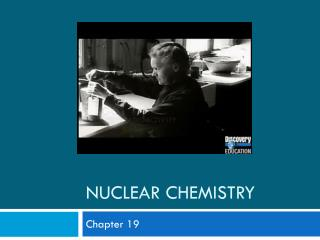# Nuclear Chemistry - PowerPoint PPT PresentationDownload PresentationNuclear Chemistry

Nuclear ChemistryDownload Presentation## Nuclear Chemistry

- - - - - - - - - - - - - - - - - - - - - - - - - - - E N D - - - - - - - - - - - - - - - - - - - - - - - - - - -
##### Presentation Transcript

1. Nuclear Chemistry Chapter 19

2. Introduction to Nuclear Chemistry • Nuclear chemistry is the study of the structure of and the they undergo. atomic nuclei changes

3. Alpha radiation • Composition – Alpha particles, same as helium nuclei • Symbol – Helium nuclei, He, α • Charge – 2+ • Mass (amu) – 4 • Penetrating power – low (0.05 mm body tissue) • Shielding – paper, clothing 4 2

4. Beta radiation • Composition – Beta particles, same as an electron • Symbol – e-, β • Charge – 1- • Mass (amu) – 1/1837 (practically 0) • Penetrating power – moderate (4 mm body tissue) • Shielding – metal foil

5. Gamma radiation • Composition – High-energy electromagnetic radiation • Symbol – γ • Charge – 0 • Mass (amu) – 0 • Penetrating power – high (penetrates body easily) • Shielding – lead, concrete

6. Alpha Decay • Example 1: Write the nuclear equation for the radioactive decay of polonium – 210 by alpha emission. Step 4: Determine the other product (ensuring everything is balanced). Step 3: Write the alpha particle. Step 2: Draw the arrow. Step 1: Write the element that you are starting with. Mass # 210 4 206 He Po Pb 84 2 82 Atomic #

7. Alpha Decay • Example 2: Write the nuclear equation for the radioactive decay of radium – 226 by alpha emission. Step 4: Determine the other product (ensuring everything is balanced). Step 3: Write the alpha particle. Step 2: Draw the arrow. Step 1: Write the element that you are starting with. Mass # 226 4 222 He Ra Rn 88 2 86 Atomic #

8. Beta Decay • Example 1: Write the nuclear equation for the radioactive decay of carbon – 14 by beta emission. Step 4: Determine the other product (ensuring everything is balanced). Step 3: Write the beta particle. Step 2: Draw the arrow. Step 1: Write the element that you are starting with. Mass # e 14 0 14 C N -1 6 7 Atomic #

9. Beta Decay • Example 2: Write the nuclear equation for the radioactive decay of zirconium – 97 by beta decay. Step 4: Determine the other product (ensuring everything is balanced). Step 3: Write the beta particle. Step 2: Draw the arrow. Step 1: Write the element that you are starting with. Mass # e 0 97 97 Zr Nb -1 40 41 Atomic #

10. Radioactivity and Half-Life Half-life is the time taken for half of a radioisotope to decay. This value is constant for that particular radioisotope. Usually questions about radioactivity involve • Half-life • The time over which the radioactivity has been measured • The quantity or intensity of the radiation.

11. Worked example 1. • A radioisotope has a half-life of 4 days. The radioisotope had an initial count rate of 800 counts per minute. What will be the count rate after 16 days? At start count rate = 800 counts min-1. After 4 days = 400 counts min-1. After 8 days = 200 counts min-1. After 12 days = 100 counts min-1. After 16 days = 50 counts min-1.

12. Worked example 2. • Thallium-208 has a half life of 3.1 minutes and decays by beta emission to form a stable isotope. • What mass of a 2.08 g sample of Tl-208 will remain unchanged after 9.3 minutes? • (b) Identify the stable isotope formed by the decay of Tl-208 by beta emission. (a) At start mass = 2.08g After 3.1 mins=1.04g After 6.2 mins=0.52g After 9.3 mins=0.26g (b) Pb-208 has been formed.

13. Calculations for you to try. • Th-234 has a half-life of 24.1 days. What mass of a 20.4g sample will remain after 96.4 days? At start mass = 20.4 g After 24.1 days mass left = 10.2 g After 48.2 days mass left = 5.1 g After 72.3 days mass left = 2.55 g After 96.4 days mass left = 1.275 g

14. Calculations for you to try. • The count rate due to carbon-14 in ancient wooden timber was found to be 100 counts per minute. A sample of modern wood had a count rate of 1600 counts per minute. Given that carbon-14 has a half life of 5570 years, calculate the age of the ancient timber. At start count rate = 1600 counts min-1. After 1 half-life = 800 counts min-1. After 2 half-life = 400 counts min-1. After 3 half-life = 200 counts min-1. After 4 half-life = 100 counts min-1. Age of timber = 4 x 5570 = 22 280 years.

15. 4. A radioisotope used in a laboratory has a half life of 6.75 hours. It had a count rate of 2000 counts per minute at 8.00 a.m. on Monday. What would be the count rate at 11 a.m. the following day? Between 8.00 a.m. and 11.00 am the next day 27 hours have passed. Number of half-lives in 27 hours = 27/6.75= 4 Count rate 2000  1000  500  250  125 counts min-1.

16. Half-Life Graph